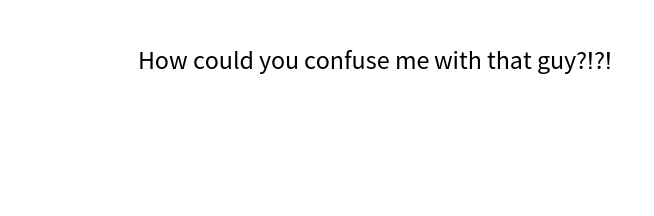#Function Repository Resource:

# SameResourceQ

Determine if resource objects are the same

Contributed by: Bob Sandheinrich
 ResourceFunction["SameResourceQ"][r1,r2,…] returns True if the resources ri represent the same ResourceObject and returns False otherwise. ResourceFunction["SameResourceQ"][r] represents an operator form of ResourceFunction["SameResourceQ"] that can be applied to resources.

## Details and Options

Resources can be specified by ResourceObject or ResourceFunction.
Resources are considered the same if they have matching UUIDs. Differing versions are ignored.
Two resource objects with the same name can be different if they are not both published in the same resource system.
ResourceFunction["SameResourceQ"][r1][r2,] is equivalent to ResourceFunction["SameResourceQ"][r1,r2,].

## Examples

### Basic Examples (3)

Specify a resource by its name and UUID and see if it is the same resource:

 In:=Out=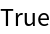See if a ResourceFunction is the same as its underlying ResourceObject:

 In:=Out=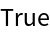A custom resource is not the same as one from the repository, even with the same name:

 In:=Out=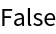### Scope (2)

Compare more than two resources:

 In:=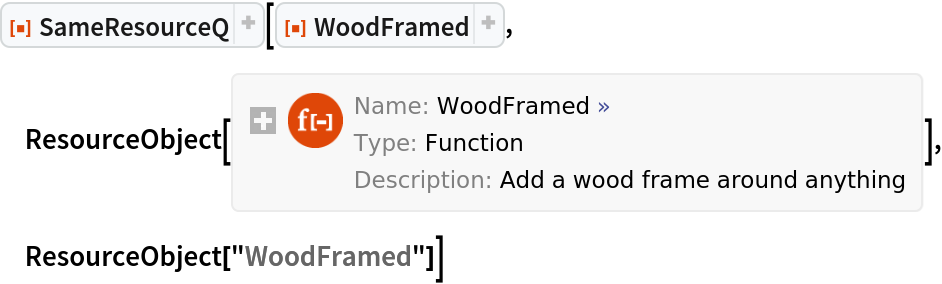Out=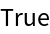Create a matching operator:

 In:=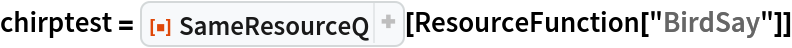Out=In:=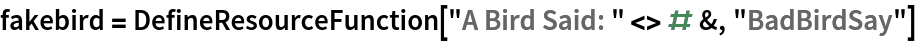Out=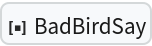In:=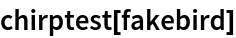Out=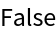In:=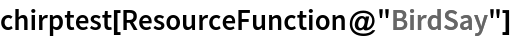Out=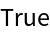### Applications (4)

After creating a resource function, the developer can end up with many saved copies:

 In:=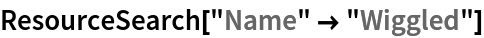Out=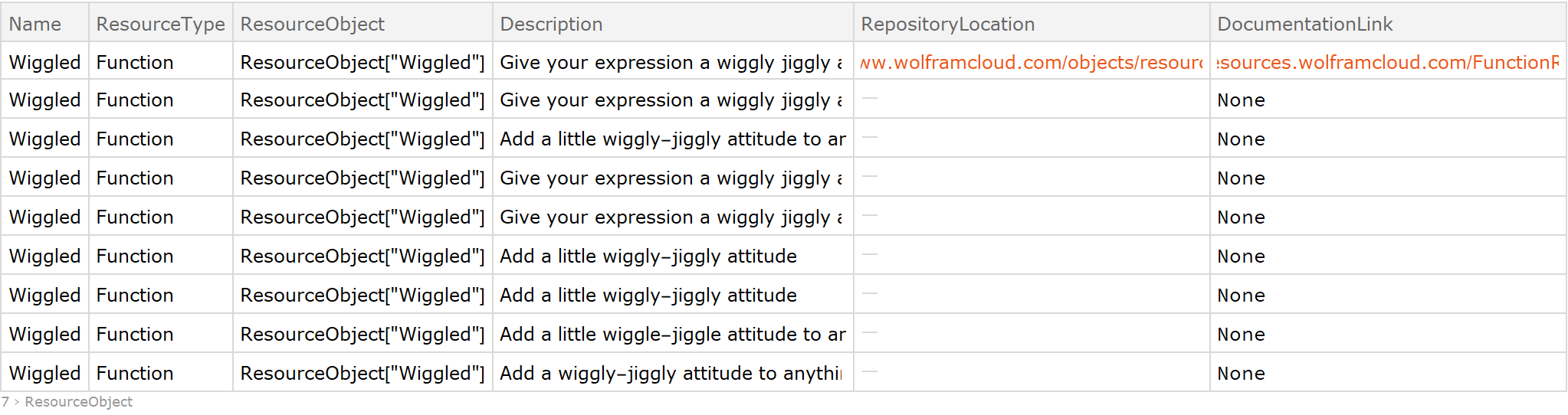Create a function to remove all but one of the deployments of a resource:

 In:=Clean up all but the published resource:

 In:=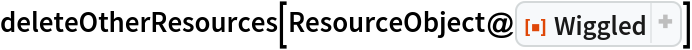Out=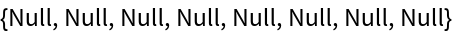Only the published version remains:

 In:=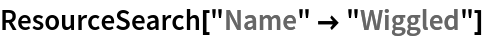Out=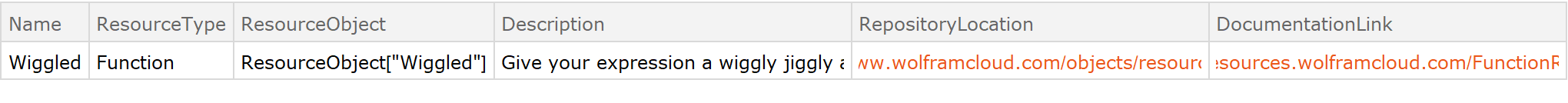### Properties and Relations (5)

SameQ often fails for ResourceObject expressions because the metadata changes when content is downloaded. Get a new resource:

 In:=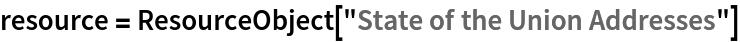Out=The content is in the cloud:

 In:=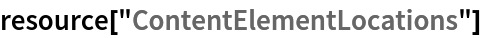Out=After retrieving the data, the resource metadata has changed:

 In:=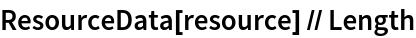Out=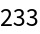In:=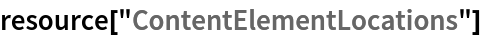Out=SameQ gives False for the output ResourceObject compared with recreating the same object:

 In:=Out=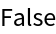SameResourceQ correctly matches the two:

 In:=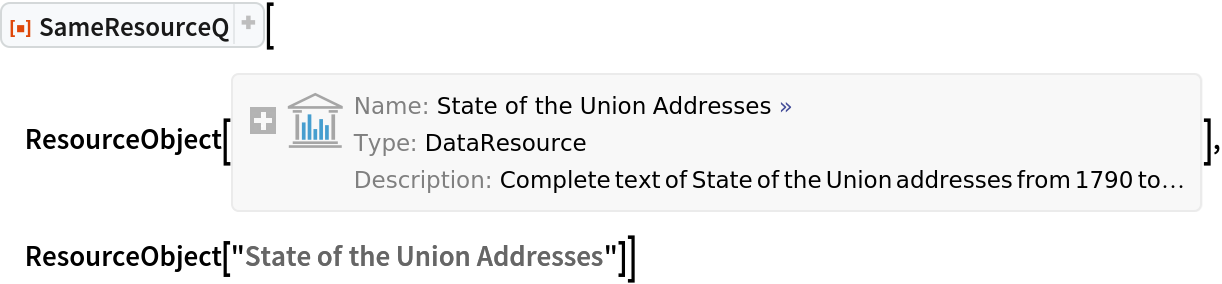Out=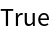### Possible Issues (2)

Resource names outside of an object are not supported:

 In:=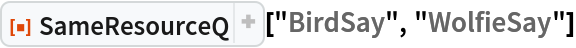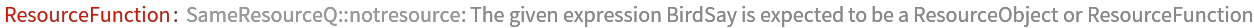Out=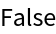In:=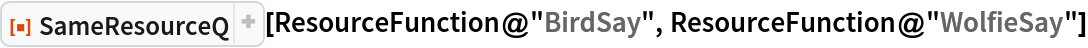Out=In:=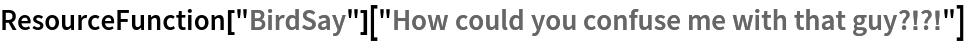Out=